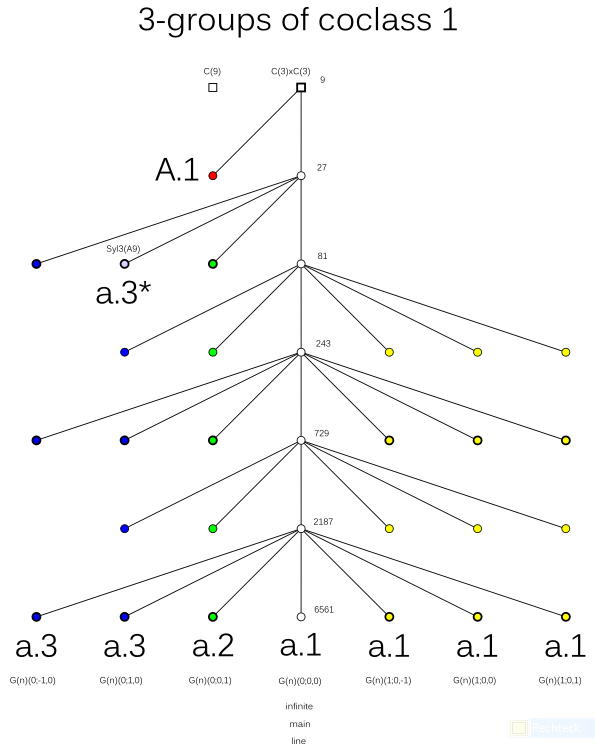# Oujda Research Project 2011

## The Coclass Graph G(3,1)

The vertices of the coclass graph G(3,1) represent all finite 3-groups G of coclass cc(G)=1.
Their abelianization G/G' is necessarily of type (3,3).
Two groups G and H are connected by a directed edge of depth one, G→H,
if G = H/N, where N denotes the last non-trivial member of the lower central series of H,
provided that N is cyclic of order 3.
Consequently, the directed edges of depth one always connect two adjacent horizontal planes
with constant orders 3n and 3n+1, differing by a factor 3.

Succinct presentation of structural details:

The two top groups (squares) are abelian. All the other groups (circles) are metabelian.
According to A. Wiman and N. Blackburn, non-metabelian p-groups of coclass 1 are impossible for p = 3.

With the aid of colors we characterize the Transfer Kernel Types (TKT), κ:
we have two sporadic groups of type A.1, κ = (1111) (red), and a.3*, κ = (2000) (cyan),
the infinite main line of type a.1, κ = (0000) (white, with abelian maximal subgroup), and coclass families
of types a.1, κ = (0000) (yellow, without abelian maximal subgroups), a.2, κ = (1000) (green), and a.3, κ = (2000) (blue).Applications in Class Field Theory:

1. Only those vertices of G(3,1) which are represented by circles with boldface contours
can be realized as second 3-class groups of real quadratic number fields K = Q(D1/2),
that is, Galois groups G32(K) = Gal(F32(K)|K) of the second Hilbert 3-class field.
They are located at depth one on the odd branches of the coclass tree T(C(3)xC(3)).

Among the 2576 real quadratic fields with discriminant 0 < D < 107 having a 3-class group of type (3,3),
the dominating part of 2303 (89.4%) has its second 3-class group G32(K) on G(3,1).
We have 697 hits of type a.3*, κ = (2000), and 1386 hits of types a.2, κ = (1000), and a.3, κ = (2000), on branch B(3) (order 81),
147 hits of type a.1, κ = (0000) without abelian maximal subgroup, and 72 hits of types a.2↑, κ = (1000), and a.3↑, κ = (2000), on branch B(5) (order 729),
and a single hit of type a.1↑, κ = (0000) without abelian maximal subgroup, on branch B(7) (order 6561).

2. For the 704 cyclic cubic number fields K with conductor 0 < f < 105 divisible by two primes
which are mutual cubic residues and such that K has a 3-class group of type (3,3)
(there are 112 further cyclic cubic fields of this kind having class number divisible by 27),
the second 3-class group G32(K) of the dominating part is abelian of type a.1, κ = (0000), and the minor part is metabelian of type A.1, κ = (1111), both on branch B(2).

 * Web master's e-mail address: contact@algebra.at *